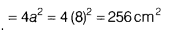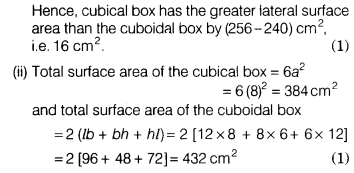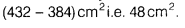# A cubical box has each edge 8 cm and another cuboidal box is 12 cm long, 8 cm

A cubical box has each edge 8 cm and another cuboidal box is 12 cm long, 8 cm wide and 6 cm high.
(i) Which box has the greater lateral surface area and by how much?
(ii) Which box has the smaller total surface area and by how much?

(i) Given, each edge of the cubical box, a = 8cm
∴ Lateral surface area of the cubical boxFor cuboidal box,
l = 12 cm, b = 8cm and h = 6cm
∴ Lateral surface area of the cuboidal boxHence, cubical box has the smaller total surface area than the cuboidal box by